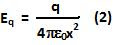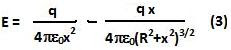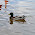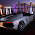## Sunday, January 24, 2010

### Irodov Problem 3.10The net electric field at a point O is the sum of the electric field Ering due to the ring and Eq due to the charge located at the center. In Problem 3.9 we have already derived Ering (in Eqn 4) as,The electric field due to the point charge at the center of the ring is given by,The net electric field is given by,We can rewrite (3) as,1.Sir, there is a problem in the Maclaurin series expansion. It should be 3 instead of 3/2
and hence, the answer will have 4 in the denominator.

2.3.The answer doesn't match to that given in the book. Plz check

4.Why is we cannot apply x>>r in the equation 3
?

5.yeah exactly...as asked by rahul anand why cant we directly neglect r in eqn 3

1.When two quantities are subtracted and variables are present, u cant neglect quantities that may hav their effect over the answer...

6.Answer is not matching with the book

7.Answer in the book is wrong